# How to Get Column Letter in Google Sheets

The guide is useful if you want to convert a column number to a column letter.

For example, column #5 in Google Sheets is also column E.

Even though the column letters are shown in the top row, there are no functions formulated solely to convert a column number to a column letter.

Unlike the `COLUMN` function, which can get a column number of a specific cell.

In this guide, we will go through two combinations of functions in Google Sheets that will enable you to convert a column number to a column letter.

Mainly:

1. `SUBSTITUTE` + `ADDRESS`
2. `REGEXEXTRACT` + `ADDRESS`

## Short Run Down on ADDRESS Function

The function returns a cell reference or address as a text or string as per the specified row and column numbers.

The way we write the `ADDRESS` function is:

`=ADDRESS(row, column, [absolute_relative_mode]) `

• The `row` is the row number of the cell reference.
• The `column` is the column number of the cell reference. For example, “A” is column number “1”.
• The `[absolute_relative_mode]` is the indicator of whether the cell reference is row or column absolute. This attribute is optional.

Here are the references for absolute relative mode:For more in-depth explanations and other examples to apply the `ADDRESS` function, do check out our article to learn more!

## Get Column Letter Using SUBSTITUTE Function

The `SUBSTITUTE` function replaces existing text with new text.

The way we write the `SUBSTITUTE` function is:

`=SUBSTITUTE(cell_to_search, search_for, replace_with) `

• The `cell_to_search` is the cell you want to make the changes to. This tells the function which cell you want it to search for to make the needed changes.
• The `search_for` is the text you want to replace. This tells the function of which text in the cell you want to change.
• The `replace_with` is the text you want to replace the `search_for` text with.

To understand the function better, don’t forget to check our article on `SUBSTITUTE` function!

Let’s combine the `SUBSTITUTE` and `ADDRESS` functions.

In this case, the `ADDRESS` function would be the `cell_to_search` attribute.

#### Example 1

1. Simply click on the cell that you want to write down your function at. In this example, it will be B2.1. Begin your function with an equal sign `=`, then followed by the name of the function, `SUBSTITUTE`, then an open parenthesis `(`.1. We will then add the `ADDRESS` function as the first attribute which is `cell_to_search`,  then add another open parenthesis `(`.1. Then add ‘1‘ as the `row` and select A2 as the `column`. Remember to insert a comma `,` in between these two attributes to separate them.1. Add another comma `,` to separate the `column` from the `absolute_relative_mode`. We then insert `'4'` as our reference, making its row and column relative. Close the `ADDRESS` function with a closing parenthesis `)`.1. We will then input ‘1‘ as the `search_for` attribute. Remember to add commas `,` to separate the attributes from each other!1. Lastly, we will insert a quote-unquote symbol `""` into the formula. By not inserting any characters in between the quote-unquote symbol `""`, we will be replacing the ‘1″ with nothing.1. After the following steps, your input should look like this:For those who are lost, no worries!

Let us explain how the formula is able to return with these values.

By just looking at the `ADDRESS` function, the return values would look like this:This is also similar to inputting the number as ‘3‘ instead of selecting A4 as the input for `column`

Moving on, by adding the `SUBSTITUTE` function, we replaced the ‘1‘ in C1. Making the end return value only showing ‘C‘.Another thing to note, we need to input `4` as the input for `absolute_relative_mode`. If we were to put either `1`, `2`, or `3`, the return value would not be only a column letter.

Here is an example to show you what happens if we input `1` as the `absolute_relative_mode`.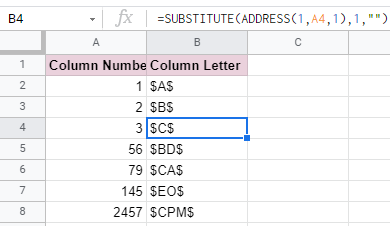The “1” would still be substituted with nothing. However, since `1` represents the “row” and “column” to return as absolute, the `\$` would appear.

## Get Column Letter Using REGEXEXTRACT Function

The `REGEXEXTRACT` function extracts a certain text string within a given data.

The way we write the `REGEXEXTRACT` function is:

`=REGEXEXTRACT(text, regular_expression)`

• The `text` is the cell where you want to match a `regular_expression` to. In our example above, it will be A2:A8.
• The `regular_expression` is the word we want to match to the text.

function is very useful, do check it out if you are interested!

Let’s combine the `REGEXEXTRACT` and `ADDRESS` functions.

In this case, the `ADDRESS` function would be the `text` attribute.

#### Example 1

1. Simply click on the cell that you want to write down your function at. In this example, it will be B2.1. Begin your function with an equal sign `=`, then followed by the name of the function, `REGEXEXTRACT`, then an open parenthesis `(`.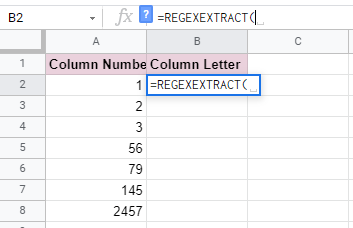1. We will then add the `ADDRESS` function as the first attribute, which is `text`.  Then add another open parenthesis `(`.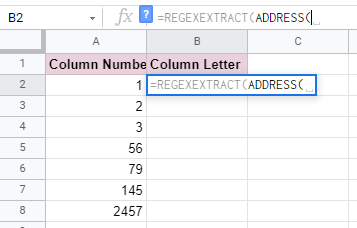1. Then add ‘1 as the `row` and select A2 as the `column`. Remember to insert a comma `,` in between these two attributes to separate them.1. Lastly, enclosed by a quote-unquote symbol `""`, type in the regular expressions we want to look for. As we only want to extract the letters, we inserted only characters ‘AZ and `+`.Take note that the `REGEXEXTRACT` function is case-sensitive. Hence, if we insert ‘a-z’ as the regular expression, it would show `#N/A`. This means the `regular_expression` we inserted could not be found in the “text”.We have also added a `+` to signify that at least one `regular_expression` is required in the `text` or additional are optional.

If we do not include a `+`, it would only extract the first letter in the `text`. As a result, this would make the return value look like this:1. After the following steps, your input should look like this:You may make a copy of the spreadsheet using the link attached below and try it for yourself:

Well done! Now you can easily convert column numbers into column rows!

Be sure to check out our tutorials on other functions that use regular expressions such as `REGEXEXTRACT`, `REGEXMATCH`, and `REGEXREPLACE`. These tutorials will provide you much more thorough and extensive description of how a regular expression works!Our goal this year is to create lots of rich, bite-sized tutorials for Google Sheets users like you. If you liked this one, you'll love what we are working on! Readers receive ✨ early access ✨ to new content.

##### You May Also Like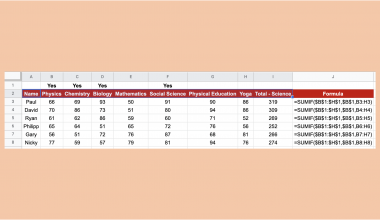## How to Use the SUMIF Function Horizontally in Google Sheets

The SUMIF function in Google Sheets is useful to get the sum of cells that meet the provided…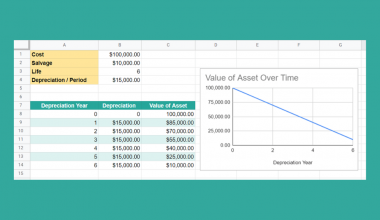## How To Use SLN Function in Google Sheets

The SLN function in Google Sheets is useful for calculating the depreciation of an asset in each period…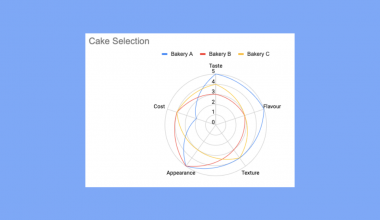Learning how to create a radar chart in Google Sheets is useful when comparing several variables quickly in…## How to Find Duplicates in New Lines Inside Cells in Google Sheets

This guide will explain how to find duplicates in new lines inside cells in Google Sheets. When working…